# Exercise 1.3 Number Systems NCERT Solutions Class 9

## Chapter 1 Ex.1.3 Question 1

Write the following in decimal form and say what kind of decimal expansion each has:

i) \begin{align}\frac{36}{100}\end{align}

ii) \begin{align}\frac{1}{11}\end{align}

iii)  \begin{align}4 \frac{1}{8}\end{align}

iv)\begin{align}\frac{3}{13}\end{align}

v) \begin{align}\frac{2}{11}\end{align}

vi) \begin{align}\frac{329}{400}\end{align}

### Solution

Steps:

(i) \begin{align}\frac{{36}}{{100}}=0.36\end{align}

Terminating decimal.

(ii) \begin{align}\frac{1}{11}\end{align}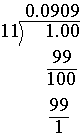The remainder $$1$$ keeps repeating. \begin{align}\frac{1}{{11}} = 0.0909\end{align} and can be written as

\begin{align}\frac{1}{{11}} = 0.\overline {09} \end{align}

Non-terminating recurring decimal.

(iii)  \begin{align}4 \frac{1}{8}=\frac{33}{8}\end{align}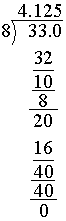\begin{align}4\frac{1}{8} = 4.125\end{align}

Terminating decimal ($$∵$$ The remainder is zero)

(iv) \begin{align}\frac{3}{13}=0.23076923\end{align}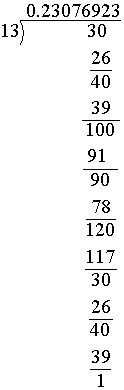$$\because$$ We find the block of numbers $$230769$$ keep repeating.

This is non-terminating recurring decimal and is written as:

\begin{align}\,\frac{3}{{13}} = 0.\overline {230769} \end{align}

(v) \begin{align}\frac{2}{{11}} = 0.1818\end{align}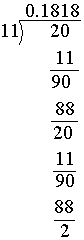Here we find the block of numbers $$18$$ keep repeating. Hence this is a non-terminating recurring decimal and is written as:

\begin{align}~\frac{2}{11}=0.\overline{18}\end{align}

(vi) \begin{align}\frac{{329}}{{400}} = \frac{{329}}{{4 \times 100}}\end{align}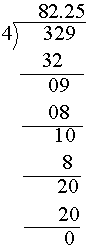\begin{align}{\frac{82.25}{100}} \\ {=0.8225}\end{align}

Terminating decimal (∵ The remainder is zero)

## Chapter 1 Ex.1.3 Question 2

You know that \begin{align}\frac{1}{7} = \overline {0.142857} .\end{align} Can you predict what the decimal expansions of \begin{align}\frac{2}{7},\frac{3}{7},\frac{4}{7},\frac{3}{7},\frac{4}{7},\frac{5}{7},\frac{6}{7}\end{align} are, without actually doing the long division? If so, how?

### Solution

What is Known?

The decimal expansion of \begin{align}\,\frac{1}{7}\end{align}.

What is Unknown?

The decimal expansions of \begin{align}\,\,\frac{2}{7},\frac{3}{7},\frac{4}{7},\frac{5}{7},\frac{6}{7}.\end{align}

Steps:

\begin{align}\frac{1}{7}=0 . \overline{142587}\end{align}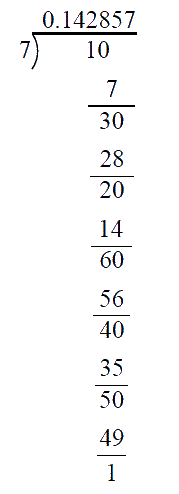This is a non-terminating recurring decimal.

We can use this to find the decimal expansion of \begin{align}\frac{2}{7},\,\,\frac{3}{7},\,\frac{4}{7},\,\frac{5}{7},\frac{6}{7}.\end{align}

To write the decimal expansion for:

i) \begin{align}\frac{2}{7}: \end{align} We observe that we get $$2$$ as remainder after the second step in the above division.

Hence,we start writing the quotient after the second decimal place and we get \begin{align}\frac{2}{7} = 0.\overline {285714} \end{align}

ii) \begin{align}\frac{3}{7}:\end{align} $$3$$ is the remainder after the first step.

\begin{align}\rm{Hence}\; \frac{3}{7}=0.\overline {428571}\end{align}

iii) \begin{align}\frac{4}{7}:\end{align} $$4$$ is the remainder at the $$4^{th}$$ step.

\begin{align}\text{Hence }\frac{4}{7}=0.\overline {571428} \end{align}

iv) \begin{align}\frac{5}{7}:\end{align}$$5$$ is the remainder at the $$5^{th}$$ step

\begin{align}\text{Hence }\frac{5}{7} = 0.\overline {714285} \end{align}

v)\begin{align}\frac{6}{7}:\end{align} $$6$$ is the remainder after the $$3^{rd}$$ step.

\begin{align}\text{Hence }\frac{6}{7} = 0.\overline {857142} \end{align}

## Chapter 1 Ex.1.3 Question 3

Express the following in the form of \begin{align}\frac{\mathrm{p}}{\mathrm{q}},\end{align} where $$p$$ and $$q$$ are integers and $$q≠ 0$$.

(i) \begin{align}0.\overline 6\end{align}

(ii) \begin{align}0.4\overline 7\end{align}

(iii) \begin{align} 0.\overline {001} .\end{align}

### Solution

Steps:

\begin{aligned} \text { (i) } &0 . \overline{6} \\ & \text { Let } \mathrm{x} =0 . \overline{6} \\&\qquad \mathrm{x} =0.666 \ldots \qquad \qquad \dots(1) \end{aligned}

Since one digit is repeating. We should multiply both sides of ($$1$$) by $$10$$. We get,

\begin{align}10\,{\rm{ x}} &= {\rm{ }}6.666...\\&= {\rm{ }}6{\rm{ }} + {\rm{ }}0.666...\\10\;{\rm{ x}}& = {\rm{ }}6{\rm{ }} + {\rm{ }}x\\10{\rm{\,x }}{\rm{ }}-{\rm{x }}{\rm{ }} &= {\rm{ }}6\\9\,{\rm{x }} &= {\rm{ }}6\\\rm{x} &= \frac{6}{9}\\&= \frac{2}{3}\\\end{align}

Hence\begin{align}0.\overline 6 = \frac{2}{3}\end{align}

\begin{align}{\rm{(ii}})\quad 0.4\overline 7 \end{align}

\begin{align}\text{Let x = 0.4777} \qquad…… (1) \end{align}

Here the repetition starts after the first decimal place and one digit is repeated.

\begin{align}\text{10 x} = 4.777 \qquad…... (2)\end{align}

\begin{align}(2) – (1)\; \text{gives}\end{align}

\begin{align} 10 \mathrm{x}-\mathrm{x} &=4.777 \ldots-0.4777 \ldots \\ 9 \mathrm{x} &=4.3 \\ 9 \mathrm{x} &=\frac{43}{10} \\ ∴ \mathrm{x} &=\frac{43}{90} \end{align}

Hence \begin{align}0.4 \overline{7}=\frac{43}{90}\end{align}

\begin{align}\rm(iii) \quad 0.\overline {001} \end{align}

\begin{align}\text{Let x }= 0.001001…… \qquad(1)\end{align}

Since $$3$$ digits are repeated multiply both the sides of ($$1$$) by $$1000$$

\begin{align}1000\;\mathrm{x}&=1.001001 \\ 1000\;\mathrm{x}&=1+0.001001 \\ 1000 \;\mathrm{x}&=1+\mathrm{x} \\ 1000\;\mathrm{x}-\mathrm{x}&=1 \\ 999 \;\mathrm{x}&=1 \\ ∴ \;\mathrm{x}&=\frac{1}{999}\end{align}

## Chapter 1 Ex.1.3 Question 4

Express \begin{align} 0.99999…\end{align} in the form of \begin{align}\frac{p}{q}\end{align}. Are you surprised with your Answer? With your teacher and classmates discuss why the answer makes sense?

### Solution

Steps:

Let  \begin{align}\text{ x }= 0.99999…...\qquad (1)\end{align}

Since one digit is repeated. We should multiply both the sides of $$(1)$$ by $$10$$

\begin{align}10\;\mathrm{x}&=9.9999 \\ 10\;\mathrm{x}&=9+0.9999 \\ 10\;\mathrm{x}&=9+\mathrm{x} \\ 10 \;\mathrm{x}-\mathrm{x}&=9 \\ 9\; \mathrm{x}&=9 \\ \mathrm{x}&=1\end{align}

Hence $$0.99999 = 1$$

## Chapter 1 Ex.1.3 Question 5

What can be the maximum number of digits be in the repeating block of digits in decimal expansion of \begin{align}\,\frac{1}{{17}}\end{align}? Perform the division to check your Answer.

### Solution

Steps:

Let us perform the division \begin{align}1÷ 17\end{align}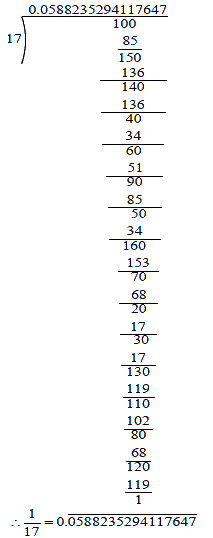There are $$16$$ digits in the repeating block of the decimal expansion of \begin{align}\frac{1}{{17}}\end{align}.

## Chapter 1 Ex.1.3 Question 6

Look at the several examples of rational numbers in the form \begin{align}\frac{p}{q}\end{align} \begin{align}(q \ne 0)\end{align} where $$p$$ and $$q$$ are integers with no common factors other than $$1$$ and having terminating decimal representation (expansions). Can you guess what property $$q$$ must satisfy?

### Solution

Steps:

We shall look at some examples of rational numbers in the form of \begin{align}\frac{{\rm{p}}}{{\rm{q}}}\end{align} \begin{align}(q \ne 0)\end{align} where decimal representations are terminating.

\begin{align}\frac{2}{5} &= 0.4 \qquad \qquad\frac{3}{{100}} = 0.03\\\\\frac{{27}}{{16}} & = 1.6875 \qquad \quad \frac{{33}}{{50}} = 0.66\end{align}

We observed that the denominators of above rational numbers are in the form of \begin{align}{2^a} \times {5^b}\end{align} Where, $$a$$ and $$b$$ are whole numbers.

Hence if $$q$$ is in the form \begin{align}{2^a} \times {5^b}\end{align} then \begin{align}\frac{{\rm{p}}}{{\rm{q}}}\end{align} is a terminating decimal.

## Chapter 1 Ex.1.3 Question 7

Write three numbers whose decimal expansions are non–terminating and non-recurring.

### Solution

Steps:

(i) $$0.212212221…$$

(ii) $$0.03003000300003…$$

(iii) $$0.825882588825… \ldots$$

## Chapter 1 Ex.1.3 Question 8

Find three irrational numbers between the rational numbers \begin{align}\frac{5}{7}\end{align} and \begin{align}\,\frac{9}{{11}}\end{align}.

### Solution

Steps:

Let us find the decimal expansion of \begin{align}\frac{5}{7}\end{align} and \begin{align}\frac{9}{{11}}\end{align} .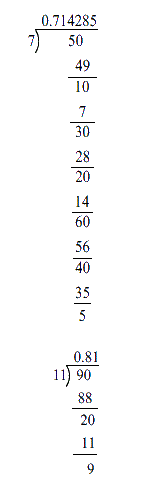We can write $$3$$ irrational numbers between them as follows:

(i) \begin{align}0.723350980011234...\end{align}

(ii) \begin{align} 0.77653472899423…\end{align}

(iii) \begin{align}0.80983435253947…\end{align}

## Chapter 1 Ex.1.3 Question 9

Classify the following numbers as rational or irrational:

(i) \begin{align}\sqrt{23}\end{align}

(ii) \begin{align} \sqrt{225}\end{align}

(iii) \begin{align} 0.3796\end{align}

iii) \begin{align}0.3796\end{align}

iv) \begin{align}7.478478 \ldots . . . .=7. \overline{478}\end{align}

v) \begin{align}1.101001000100001\end{align}

### Solution

Steps:

i) \begin{align}\sqrt {23} = \frac{{\sqrt {23} }}{1} = \frac{p}{q},\end{align} but $$p$$ is not an integer.

Hence \begin{align}{\sqrt{23}}\end{align} is an irrational number.

ii) \begin{align} \sqrt {225} = \frac{{15}}{1} = \frac{p}{q},\end{align} where $$p$$ and $$q$$ are integers. \begin{align}q \ne 0.\end{align}

Hence \begin{align}\sqrt {225} \end{align} is a rational number.

iii) \begin{align}0.3796\end{align}

\begin{align}0.3796\end{align} is a rational number . Because it is a terminating decimal number.

iv) \begin{align}7.478478 \ldots . . . .=7. \overline{478}\end{align}

It is an rational number. As It is a non-terminating recurring decimal.

v) \begin{align}1.101001000100001\end{align}

It is an irrational number because it is a non-terminating and non-recurring decimal.

Instant doubt clearing with Cuemath Advanced Math Program